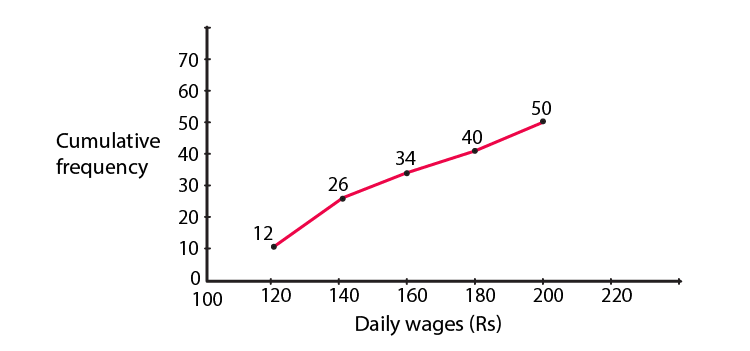# RD Sharma Solutions for Class 10 Maths Chapter 7 Statistics Exercise 7.6

Exercise 7.6 primarily discusses the relationship among mean, median and mode as well as constructing a cumulative frequency polygon(OGIVE). Problems in this exercise are well crafted to understand the two types of ogives and its applications. Students wanting to get an edge over these concepts can access the RD Sharma Solutions Class 10. Get the RD Sharma Solutions for Class 10 Maths Chapter 7 Statistics Exercise 7.6 PDF is provided below for further assistance when solving this exercise.

## RD Sharma Solutions for Class 10 Maths Chapter 7 Statistics Exercise 7.6 Download PDF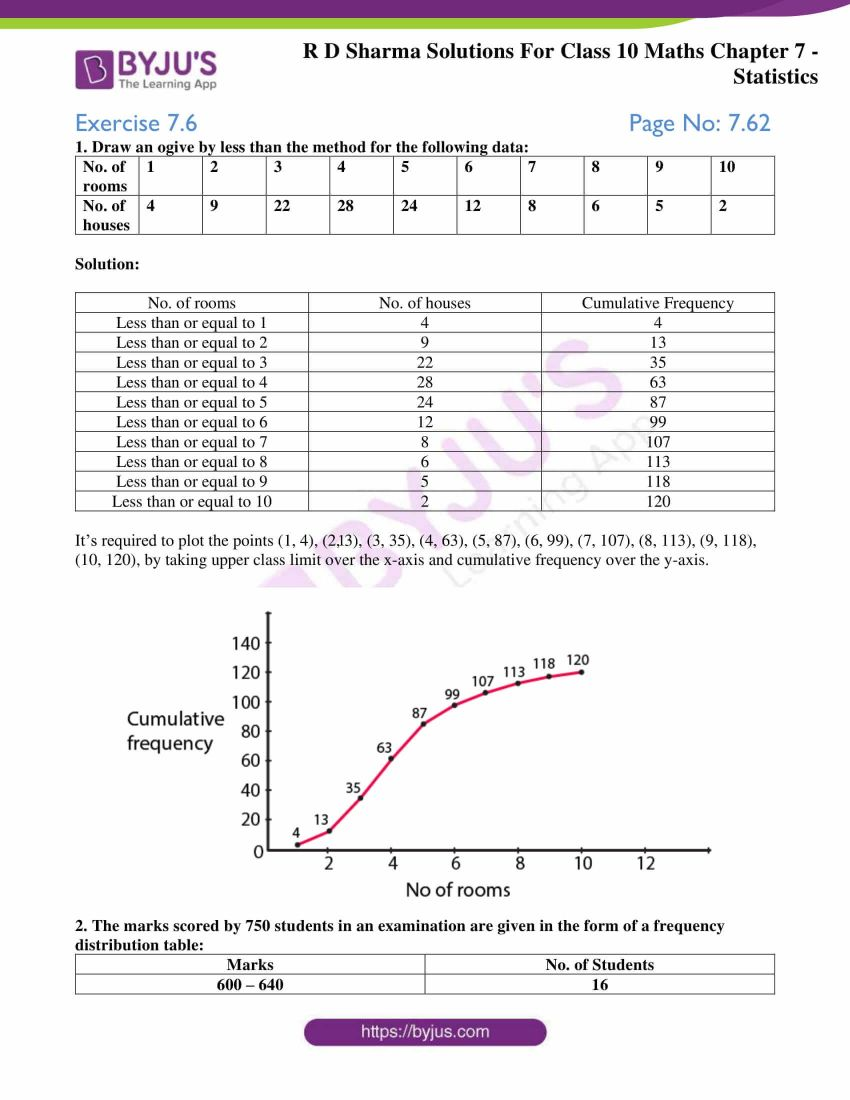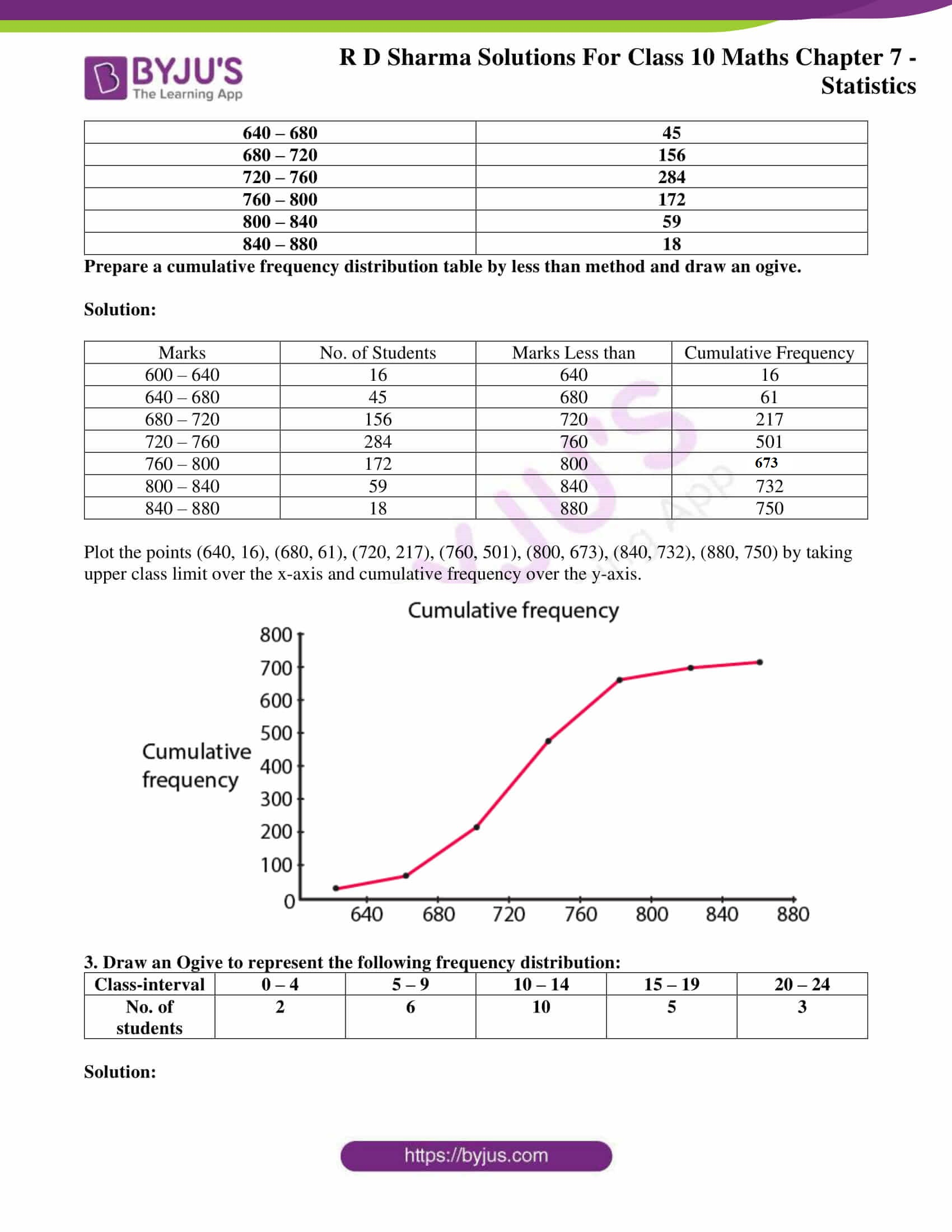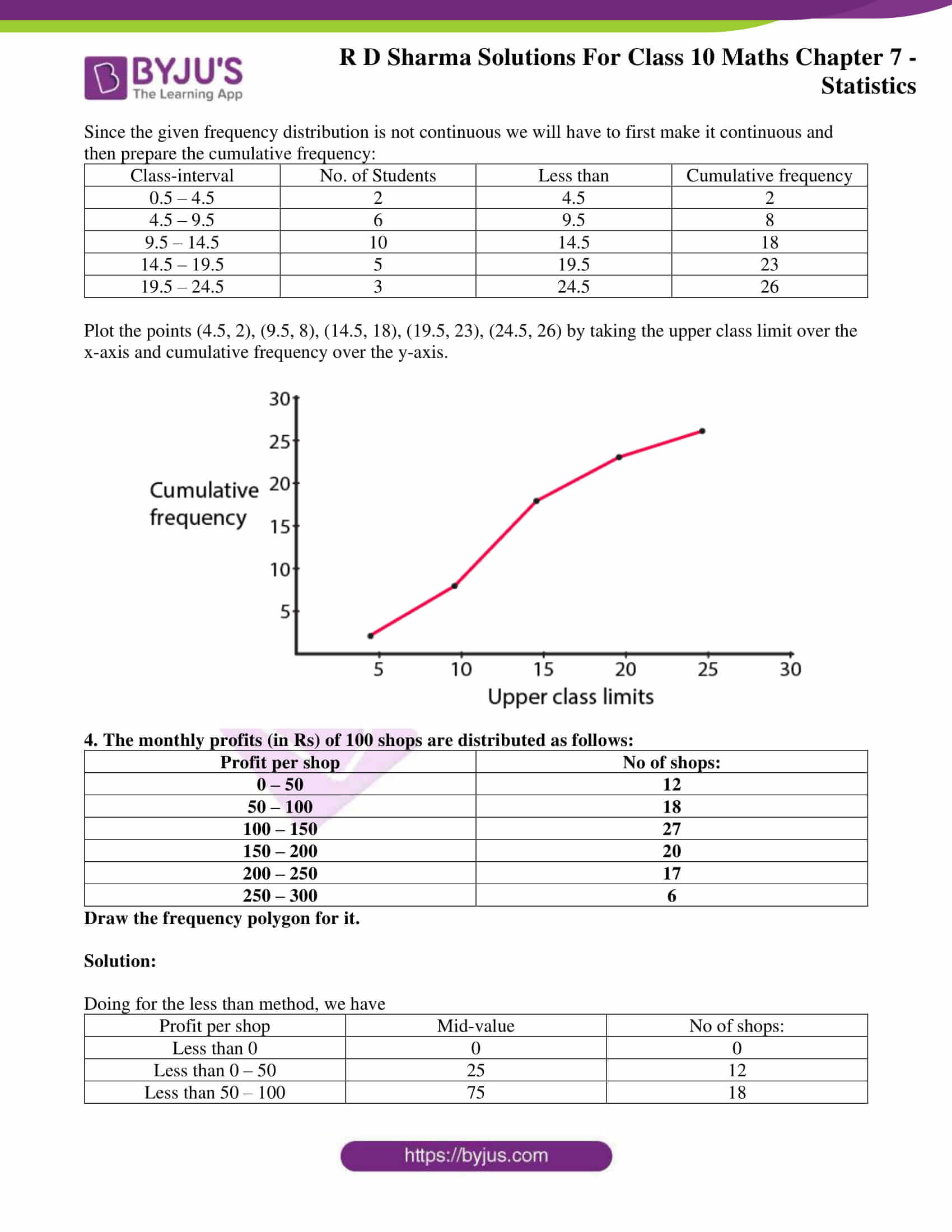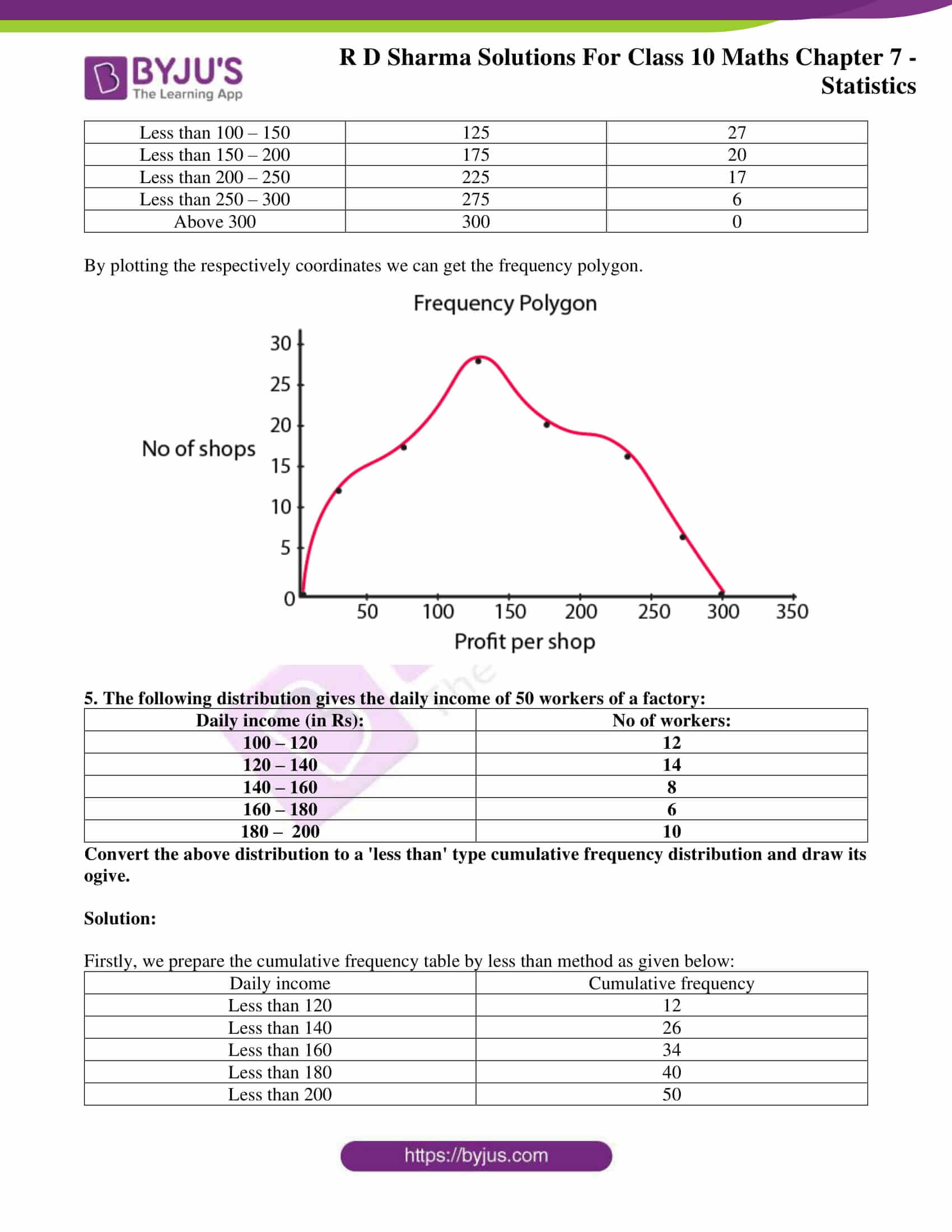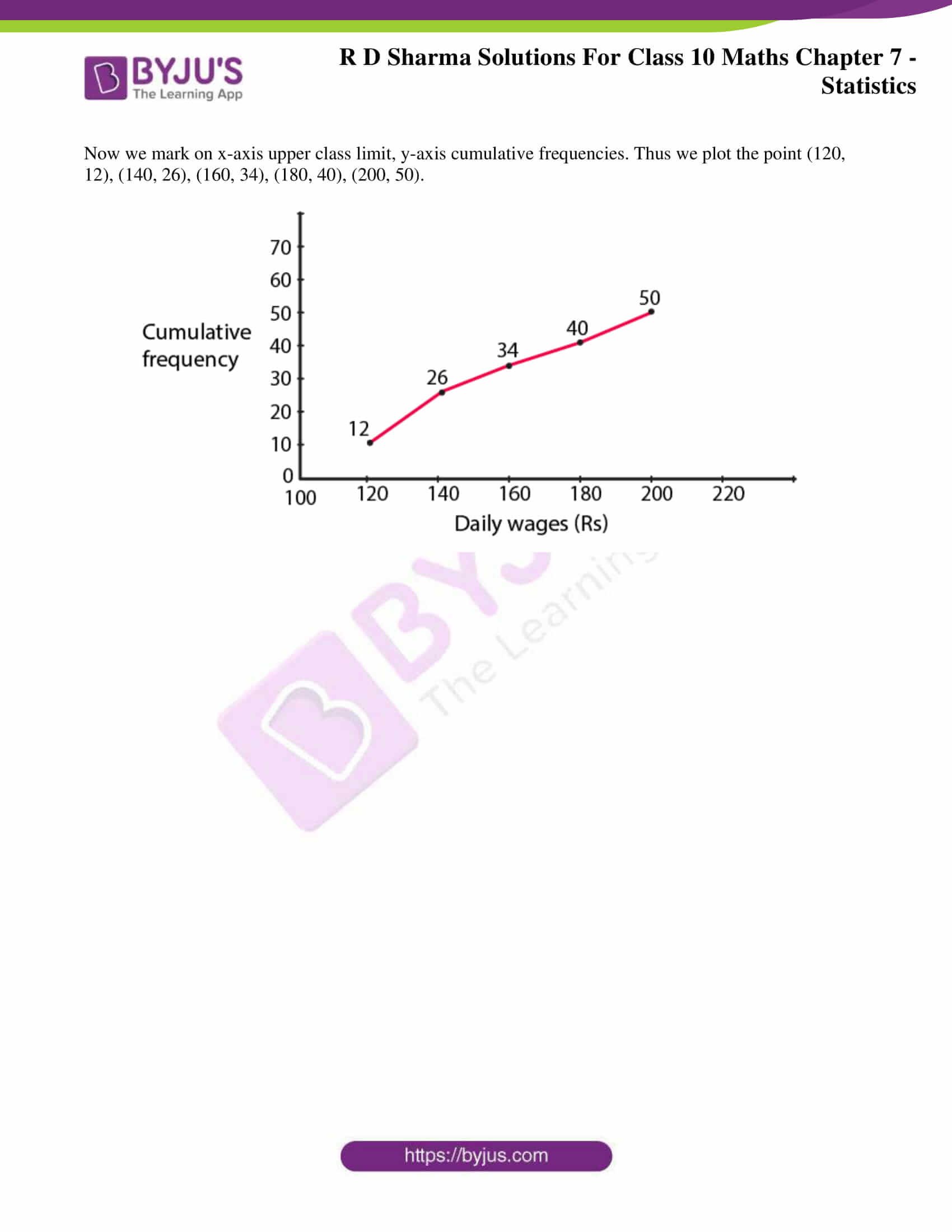### Access RD Sharma Solutions for Class 10 Maths Chapter 7 Statistics Exercise 7.6

1. Draw an ogive by less than the method for the following data:

 No. of rooms 1 2 3 4 5 6 7 8 9 10 No. of houses 4 9 22 28 24 12 8 6 5 2

Solution:

 No. of rooms No. of houses Cumulative Frequency Less than or equal to 1 4 4 Less than or equal to 2 9 13 Less than or equal to 3 22 35 Less than or equal to 4 28 63 Less than or equal to 5 24 87 Less than or equal to 6 12 99 Less than or equal to 7 8 107 Less than or equal to 8 6 113 Less than or equal to 9 5 118 Less than or equal to 10 2 120

It’s required to plot the points (1, 4), (2, 13), (3, 35), (4, 63), (5, 87), (6, 99), (7, 107), (8, 113), (9, 118), (10, 120), by taking upper-class limit over the x-axis and cumulative frequency over the y-axis.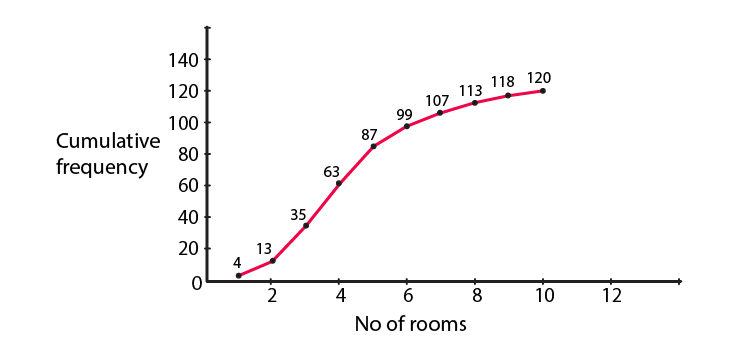2. The marks scored by 750 students in an examination are given in the form of a frequency distribution table:

 Marks No. of Students 600 – 640 16 640 – 680 45 680 – 720 156 720 – 760 284 760 – 800 172 800 – 840 59 840 – 880 18

Prepare a cumulative frequency distribution table by less than method and draw an ogive.

Solution:

 Marks No. of Students Marks Less than Cumulative Frequency 600 – 640 16 640 16 640 – 680 45 680 61 680 – 720 156 720 217 720 – 760 284 760 501 760 – 800 172 800 673 800 – 840 59 840 732 840 – 880 18 880 750

Plot the points (640, 16), (680, 61), (720, 217), (760, 501), (800, 673), (840, 732), (880, 750) by taking upper class limit over the x-axis and cumulative frequency over the y-axis.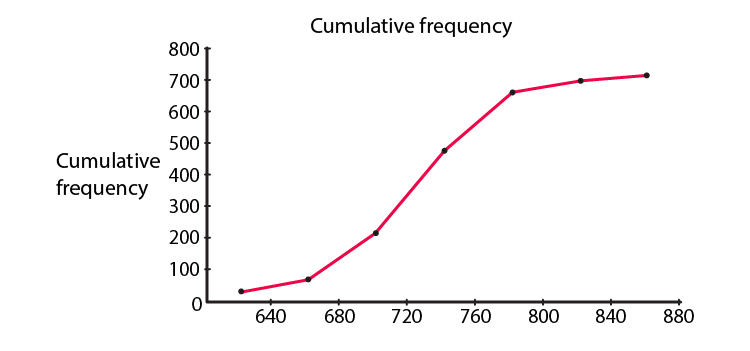3. Draw an Ogive to represent the following frequency distribution:

 Class-interval 0 – 4 5 – 9 10 – 14 15 – 19 20 – 24 No. of students 2 6 10 5 3

Solution:

Since the given frequency distribution is not continuous we will have to first make it continuous and then prepare the cumulative frequency:

 Class-interval No. of Students Less than Cumulative frequency 0.5 – 4.5 2 4.5 2 4.5 – 9.5 6 9.5 8 9.5 – 14.5 10 14.5 18 14.5 – 19.5 5 19.5 23 19.5 – 24.5 3 24.5 26

Plot the points (4.5, 2), (9.5, 8), (14.5, 18), (19.5, 23), (24.5, 26) by taking the upper class limit over the x-axis and cumulative frequency over the y-axis.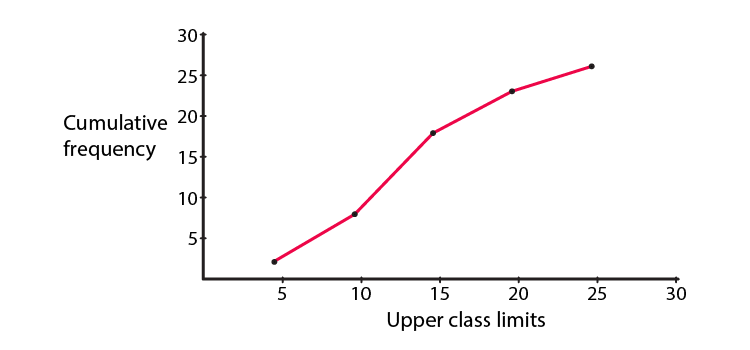4. The monthly profits (in Rs) of 100 shops are distributed as follows:

 Profit per shop No of shops: 0 – 50 12 50 – 100 18 100 – 150 27 150 – 200 20 200 – 250 17 250 – 300 6

Draw the frequency polygon for it.

Solution:

Doing for the less than method, we have

 Profit per shop Mid-value No of shops: Less than 0 0 0 Less than 0 – 50 25 12 Less than 50 – 100 75 18 Less than 100 – 150 125 27 Less than 150 – 200 175 20 Less than 200 – 250 225 17 Less than 250 – 300 275 6 Above 300 300 0

By plotting the respectively coordinates we can get the frequency polygon.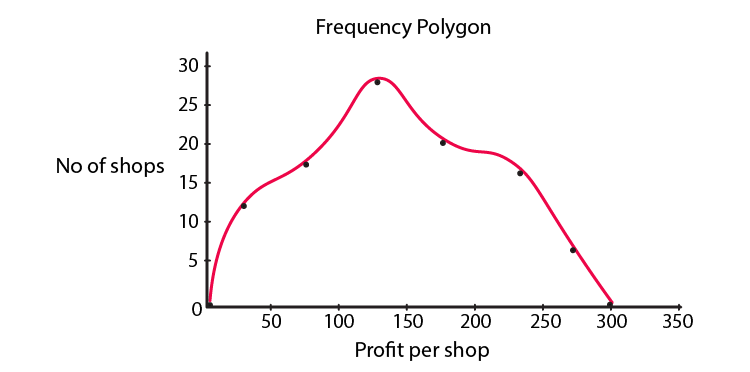5. The following distribution gives the daily income of 50 workers of a factory:

 Daily income (in Rs): No of workers: 100 – 120 12 120 – 140 14 140 – 160 8 160 – 180 6 180 – 200 10

Convert the above distribution to a ‘less than’ type cumulative frequency distribution and draw its ogive.

Solution:

Firstly, we prepare the cumulative frequency table by less than method as given below:

 Daily income Cumulative frequency Less than 120 12 Less than 140 26 Less than 160 34 Less than 180 40 Less than 200 50

Now we mark on x-axis upper class limit, y-axis cumulative frequencies. Thus we plot the point (120, 12), (140, 26), (160, 34), (180, 40), (200, 50).